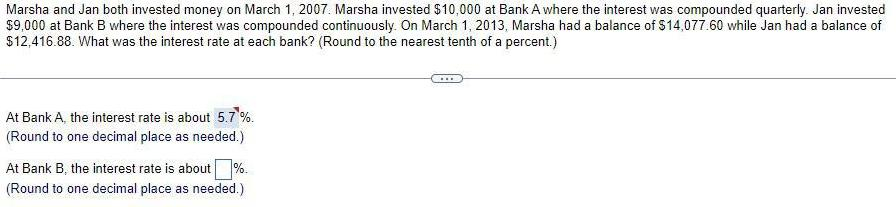Question:

# Marsha and Jan both invested money on March 1, 2007. Marsha

Last updated: 7/4/2022Marsha and Jan both invested money on March 1, 2007. Marsha invested \$10,000 at Bank A where the interest was compounded quarterly. Jan invested \$9,000 at Bank B where the interest was compounded continuously. On March 1, 2013, Marsha had a balance of \$14,077.60 while Jan had a balance of \$12,416.88. What was the interest rate at each bank? (Round to the nearest tenth of a percent.) At Bank A, the interest rate is about 5.7%. (Round to one decimal place as needed.) At Bank B, the interest rate is about %. (Round to one decimal place as needed.) ***